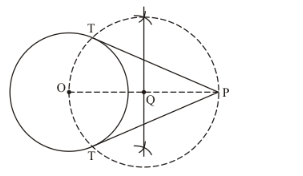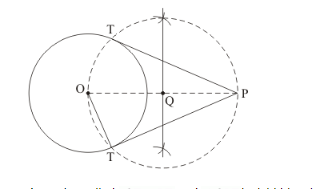# Draw a circle of radius 6 cm. From a point 10 cm away from its centre,

Question:

Draw a circle of radius 6 cm. From a point 10 cm away from its centre, construct the pair of tangents to the circle and measure their lengths.

Solution:

Given that

Construct a circle of radius, andform its centre, construct the pair of tangents to the circle.

Find the length of tangents.

We follow the following steps to construct the giventep of construction

Step: I- First of all we draw a circle of radius.

Step: II- Make a point P at a distance of, and join.

Step: III -Draw a right bisector of, intersectingat Q .

Step: IV- Taking as centre and radius, draw a circle to intersect the given circle at T and T’.

Step: V- Joins PT and PT’ to obtain the require tangents.

Thus,are the required tangents.

Find the length of tangents.As we know that $O T \perp P T$ and $\triangle O P T$ is right triangle.

Therefore,

$O T=6 \mathrm{~cm}$ and $P O=10 \mathrm{~cm}$

In $\triangle O P T$,

$P T^{2}=O P^{2}-O T^{2}$

$=10^{2}-6^{2}$

$=100-36$

$=64$

$P T=\sqrt{64}$

$=8$

Thus, the length of tangents $=8 \mathrm{~cm}$